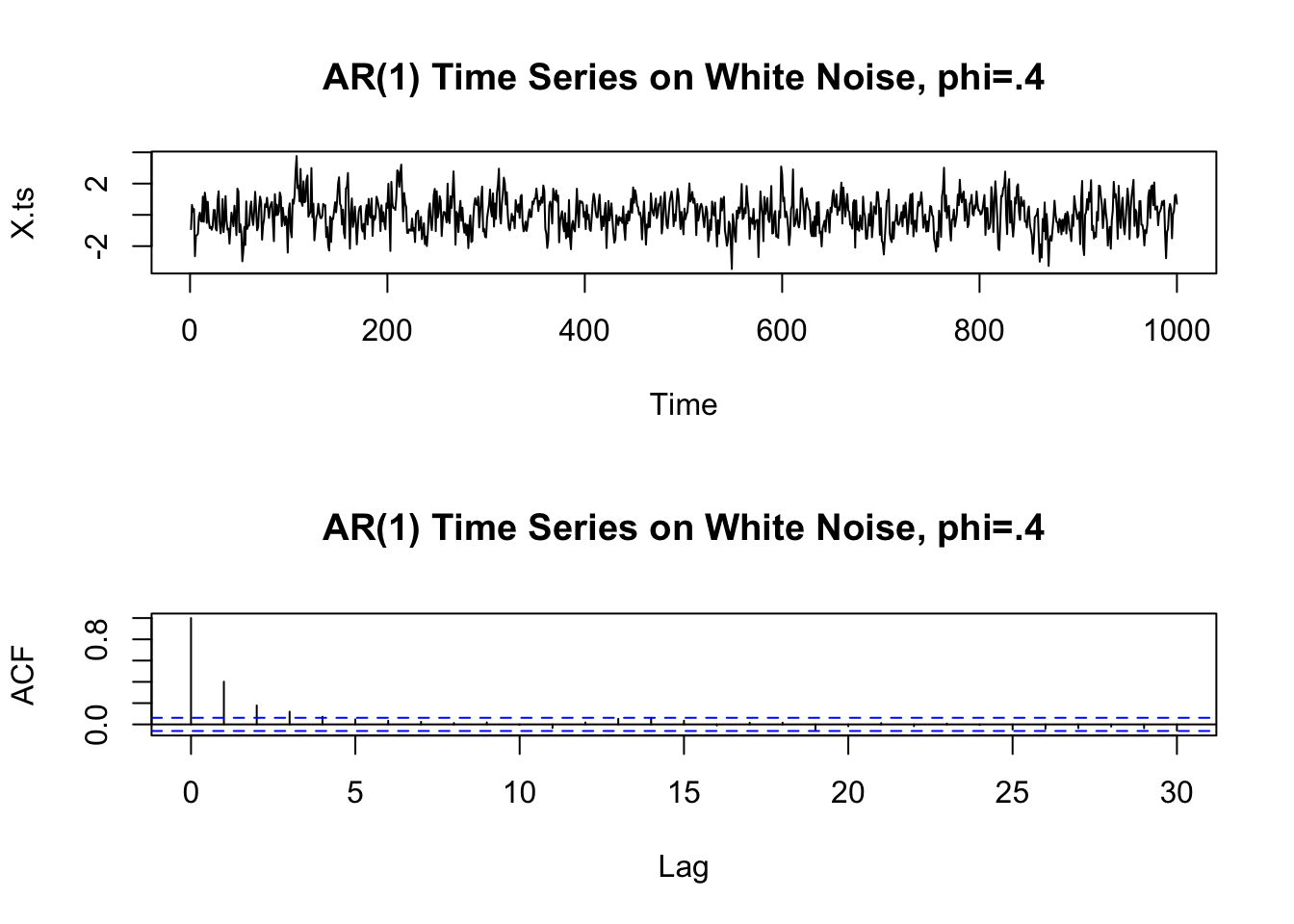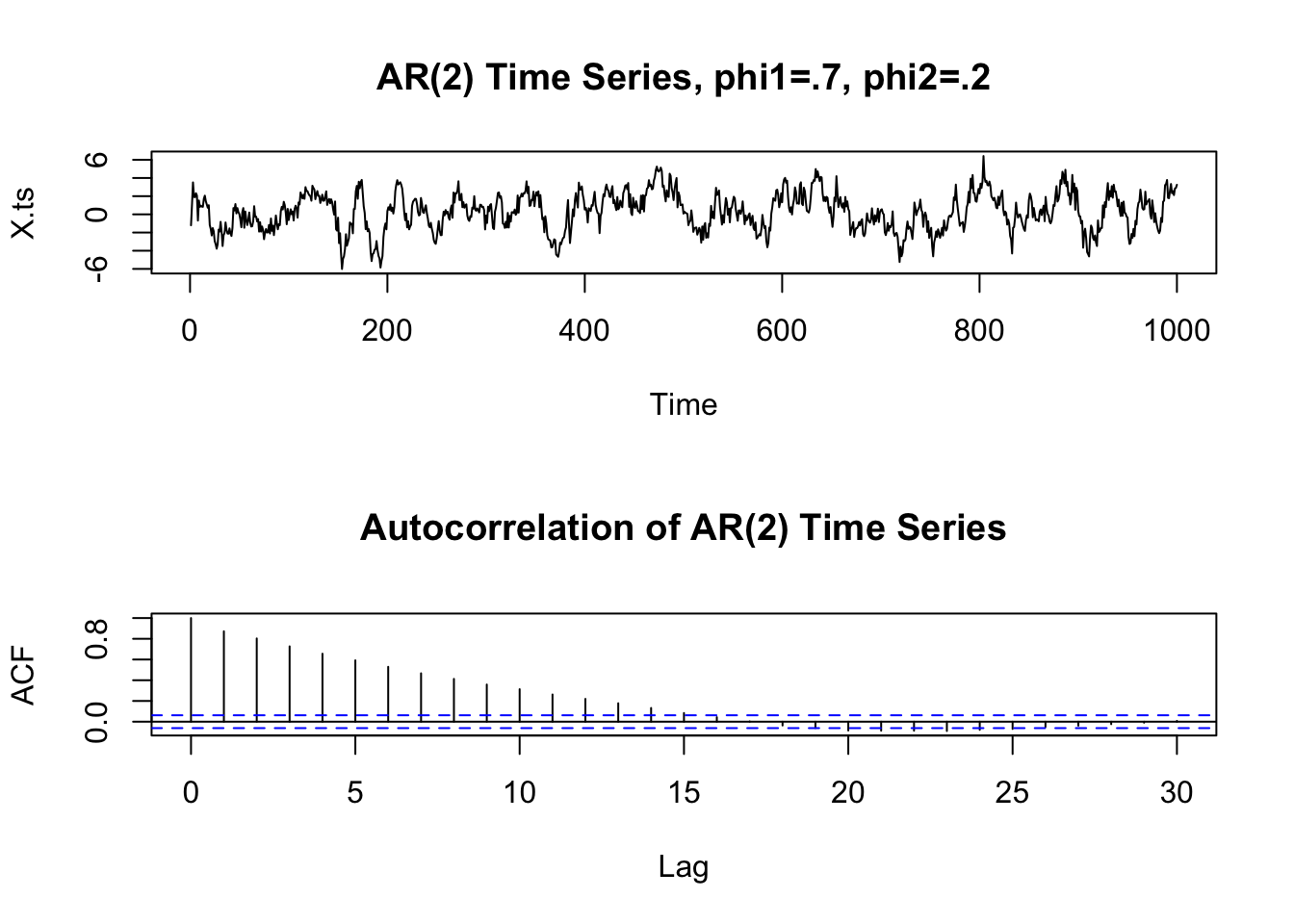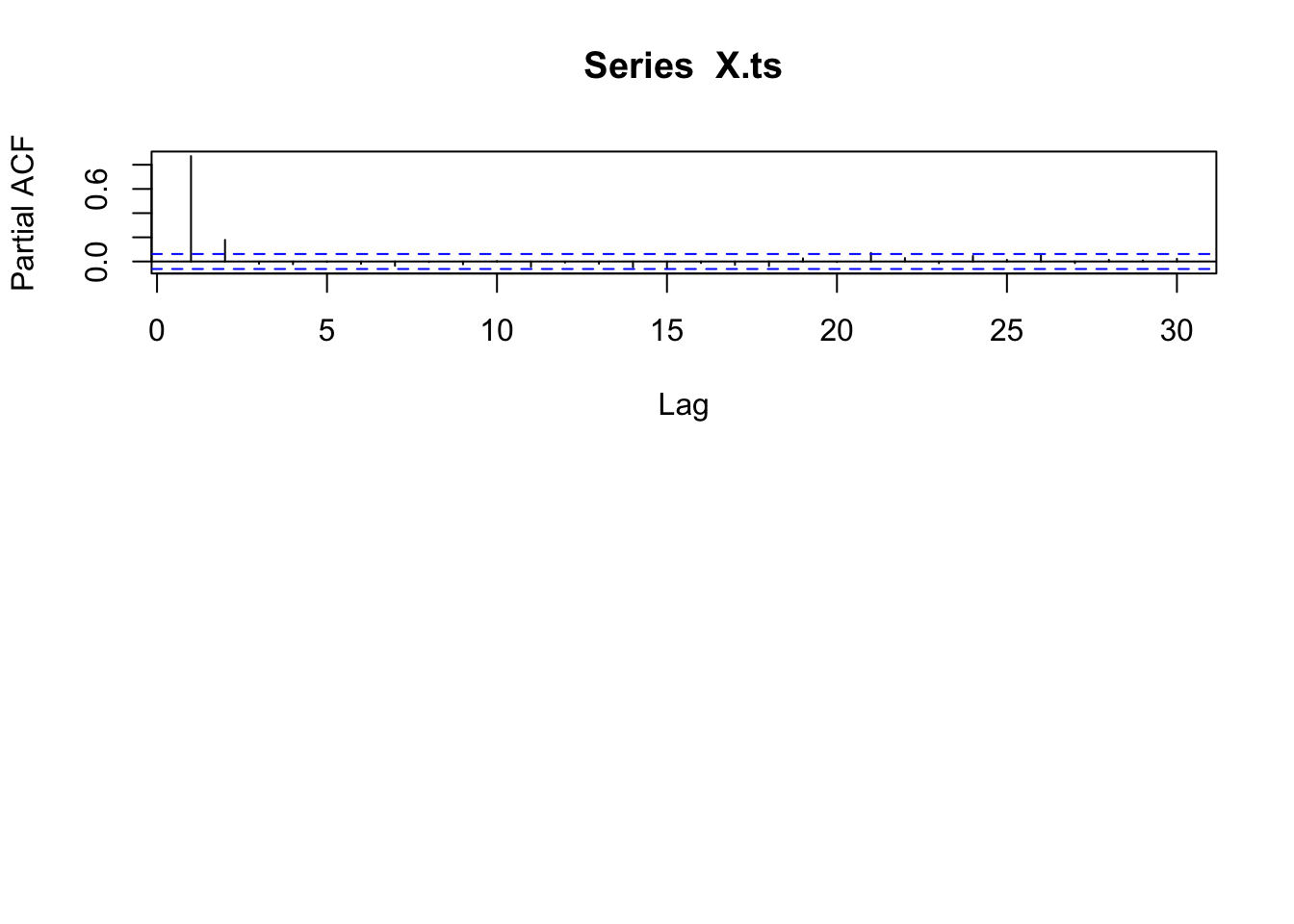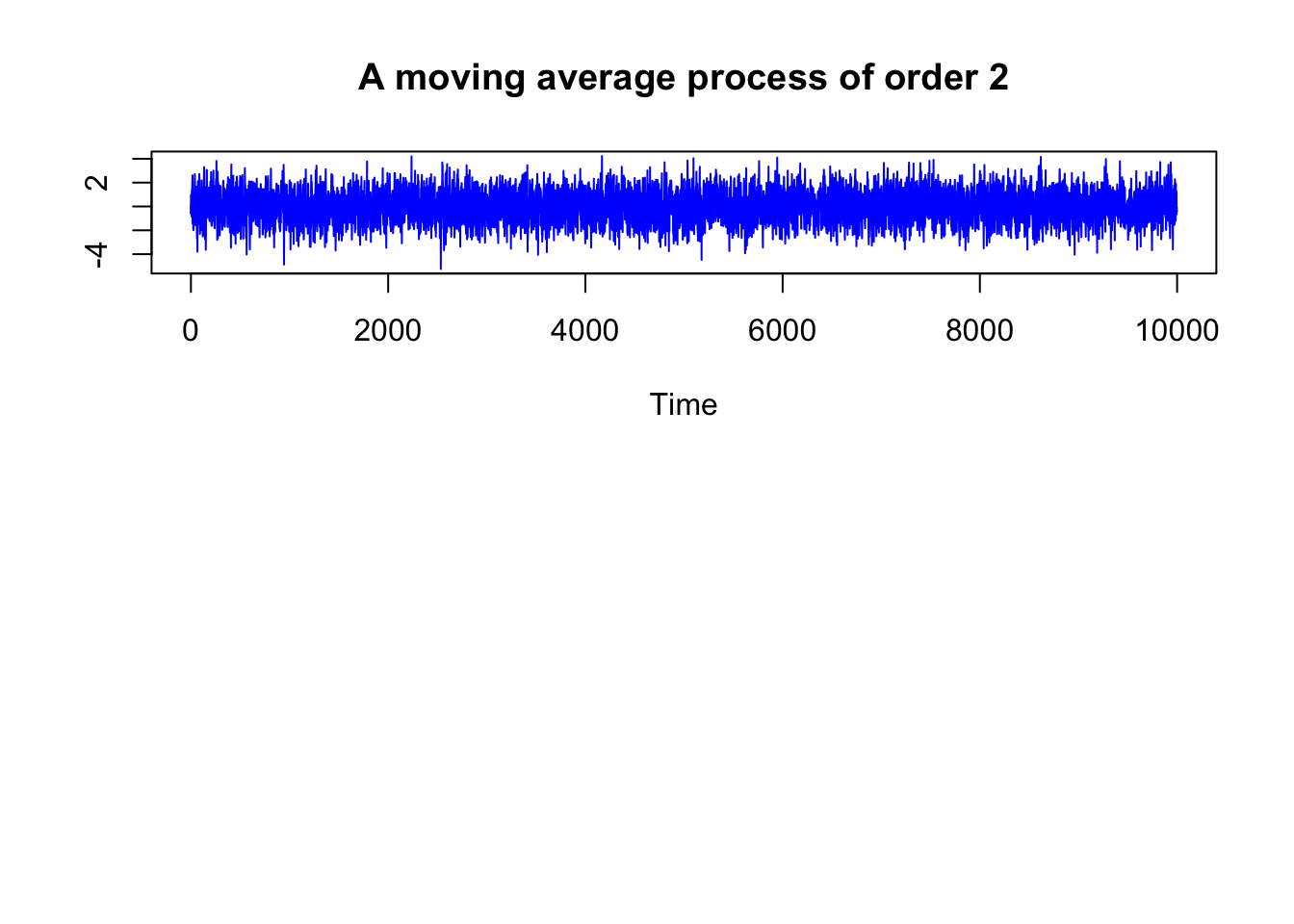# Introduction to Time Series

statistics
Author

Stefan Eng

Published

July 6, 2019

## Classical decomposition model

$X_t = m_t + s_t + y_y$ where - $$m : \mathbb{Z} \rightarrow \mathbb{R}$$ is a slowly changing function, the trend component. - $$s : \mathbb Z \rightarrow \mathbb R$$ is a function with a known period $$d$$, i.e., $$s_{t + d} = s_t$$ for all $$t \in \mathbb Z$$ and $$\sum_{j = 1}^d s_j = 0$$, is called the seasonal component. - $$(y_t, t \in \mathbb Z)$$ is a stationary stochastic process.

## Time Series Analysis

• Always plot the data first
• If there are clear sections in the data, it might be good to analyze each section separately

## Best Linear Predictor

Let $$(X_t, t \in \Z)$$ be a time series with $$Var(X_t) < \infty$$ for $$t \in \Z$$ and $$X^n := (X_{t_1},\ldots, X_{t_n})$$ be a collection of random variables of the time series at $$n$$ different times. Then the _best linear predictor of $$X_t$$ is given by $b_t^l(X^n) = a_0 + a_1 X_{t_n} + \cdots + a_n X_{t_1}$ where the coefficients are determined by the linear equations

1. $$E(X_t - b_t^l(X^n)) = 0$$
2. $$E(X_{t_j}(X_t - b_t^l(X^n))) = 0$$ for all $$j = 1,\ldots,n$$.

If X is stationary, with mean $$\mu$$ and autocovariance function $$\gamma$$, the coefficients are determined by $a_0 = \mu (1 - \sum_{i = 1}^n a_i)$ and $(\gamma(t_{n + 1 - j} - t_{n + 1 - i}))_{i,j = 1}^n (a_1,\ldots,a_n)^T = (\gamma(t - t_n),\ldots, \gamma(t - t_1))^T$

The mean square error is:

$MSE(b_t^l(X^n), X_t) = E[(b_t^l(X^n) - X_t)^2] = \gamma(0) - (a_1, \ldots, a_n)(\gamma(t - t_n), \ldots, \gamma(t - t_1))^T$

Note: when $$X^n := (X_1, \ldots, X_n)$$ then the coefficients $$(a_0,\ldots,a_n)$$ for prediction of $$X_{n + h}$$ and $(\gamma(i - j)_{i,j = 1}^n) (a_1,\ldots, a_n)^T = (\gamma(h), \ldots, \gamma(h + n - 1))^T$

### Example

The notation $$t_1,\ldots, t_n$$ was confusing to me at first. A good example that shown how it works is if we have an $$AR(1)$$ process, $X_t - \phi_1 X_{t - 1} = Z_t$ Assume that we observe values at $$X_1$$ and $$X_3$$, but are missing a value for $$X_2$$. We then have $$t_1 = 1$$, $$t_2 = 3$$, with $$n = 2$$. We want to find: $b_2^l(X^1,X^3) = a_0 + a_1 X_3 + a_2 X_1$ We have that $$a_0 = 0$$, since the process has mean zero. It then follows that,

\begin{aligned} \begin{pmatrix} \gamma(t_{3 + 1 - 1} - t_{3 + 1 - 1}) & \gamma(t_{3 + 1 - 2} - t_{3 + 1 - 1})\\ \gamma(t_{3 + 1 - 1} - t_{3 + 1 - 2}) &\gamma(t_{3 + 1 - 1} - t_{3 + 1 - 1}) \end{pmatrix} \begin{pmatrix} a_1\\ a_2 \end{pmatrix} &= \begin{pmatrix} \gamma(2 - t_{2})\\ \gamma(2 - t_{1}) \end{pmatrix}\\ \begin{pmatrix} \gamma(0) & \gamma(3 - 1)\\ \gamma(3 - 1) &\gamma(0) \end{pmatrix} \begin{pmatrix} a_1\\ a_2 \end{pmatrix} &= \begin{pmatrix} \gamma(2 - 3)\\ \gamma(2 - 1) \end{pmatrix}\\ \begin{pmatrix} 1 & \phi_1^2\\ \phi_1^2 & 1 \end{pmatrix} \begin{pmatrix} a_1\\ a_2 \end{pmatrix} &= \begin{pmatrix} \phi_1\\ \phi_1 \end{pmatrix} \end{aligned}

Which leads to a solution $a_1 = a_2 = \frac{\phi_1}{1 + \phi_1^2}$

## ARMA(p,q)

$$\{X_t\}$$ is an ARMA(p,q) process if $$\{X_t\}$$ is stationary and if for every $$t$$, $X_t - \sum_{i = 1}^p \phi_i X_{t-i} = Z_t + \sum_{j = 1}^q \theta_j Z_{t - j}$ where $$\{Z_t\} \sim WN(0, \sigma^2)$$ and the polynomials $$(1 - \sum_{i = 1}^p \phi_i z^i)$$ and $$(1 + \sum_{j = 1}^q \theta_j z^j)$$ have no common factors.

## Causality

An ARMA(p,q) process $$\{X_t\}$$ is causal, if there exists constants $$\{\psi_t\}$$ such that $\sum_{j = 0}^\infty | \psi_{j} | < \infty$ and $X_t = \sum_{j = 0}^\infty \psi_{j} Z_{t - j}$ for all $$t$$. That is, if we can represent the ARMA(p,q) process $$\{X_t\}$$ as a $$MA(\infty)$$ process.

How to actually check? Use the equivalent condition: $\phi(z) = 1 - \sum_{i = 1}^p \phi_i z^i \not = 0 \quad \text{for all}~|z| \leq 1$ That is, that $$\phi(z)$$ has no roots inside (or that all root are outside the unit circle).

### Finding Causal Representation

To represent our (causal) ARMA(p,q) process in the form: $X_t = \sum_{j = 0}^\infty \psi_j Z_{t - j}$ The sequence of $$\{\psi_j\}$$ is determined by the relation $$\psi(z) = \sum_{j = 0}^\infty \psi_j z^j = \theta(z) / \phi(z)$$, or equivalently: $\psi_j - \sum_{k = 1}^p \phi_k \psi_{j - k} = \theta_j, ~j = 0,1,\ldots$ with $$\theta_0 := 1, \theta_j := 0$$ for j > q and $$\psi_j := 0$$ for $$j < 0$$.

### Finding invertible (AR($$\infty$$)) representation

To represent our invertible ARMA(p,q) process in the form: $Z_t = \sum_{j = 0}^\infty \pi_j X_{t - j}$ we find $$\{\pi_j\}$$ by the equations $\pi_j + \sum_{k = 1}^q \theta_k \pi_{j - k} = -\phi_j, ~j = 0,1,\ldots,$ where $$\phi_0 := -1,~ \phi_j := 0$$ for $$j > p$$, and $$\pi_j := 0$$ for $$j < 0$$.

## Model Building for ARMA processes

• Remove trend and seasonality until you believe the dta can be modeled as a stationary time series.
• Identify the order of the ARMA process for the time series
• Either look at ACF/PACF
• or by fitting (using maximum likelihood or Hannan-Rissanen estimation) sucessively higher order ARMA(p,q) to the data and choosing p, q to minimize either the AICC or BIC.
• Estimate the final model using maximum likelihood
• Compute the residuals $$\hat{R}_t$$ and check that they are consistent with the specified distribution and temporal covariance structure for $$Z_t$$.
• If they are, then the model is considered adequate for the data.

## Autoregressive Processes - AR(p)

$X_t - \sum_{j = 1}^p \phi_j X_{t - j} = Z_t$

• AR(p) is not necessarily stationary!

### AR(1) Example### AR(2)

Can simulate using the function astsa::arima.sim function $X_t = Z_t + 0.7 \cdot X_{t - 1} + 0.2 \cdot X_{t - 2}$## Moving Average Processes - MA(q)

$X_t = Z_t + \sum_{j = 1}^q \theta_j Z_{t - j}$ with $$Z_t \sim WN(0, \sigma^2)$$. At lag greater than or equal to q, the ACF should be zero.

### MA(2) example

$X_t = Z_t + 0.8 \cdot Z_{t - 1} + 0.2 \cdot Z_{t - 2}$The autocorrelation function## ARMA(p,q)

• The partial autocorrelation function PACF of an ARMA(p,q) process X can be thought of as the correlation between $$X_t$$ and $$X_{t + h}$$ when adjusting for the intervening observations $$X_{t + 1}, \ldots, X_{t + h - 1}$$.
• Can be used to detect seasonality: If you have monthly data and you see a big spike at $$\hat{\alpha}(12)$$, it is likely that you see a periodic effect corresponding to a calendar year, so you should remove the seasonality.

### ACF and PACF

Conditional Mean Model ACF PACF
AR(p) Tails off gradually Cuts off after p lags
MA(q) Cuts off after q lags Tails off gradually

Source: https://se.mathworks.com/help/econ/autocorrelation-and-partial-autocorrelation.html

## Innovations Algorithm

Used to compute the best linear predictor, $$b_{n + 1}^l(X^n)$$ more computationally efficiently than solving a system of $$n$$ linear equations. Can be applied to all time series with finite second moments. Assume we have a time series $$(X_t, t \in \mathbb Z)$$ with zero mean, and finite second moment $$E[X_t^2] < + \infty$$ for all $$t \in \mathbb Z$$ and covariance $Cov(X_i, X_j) = \kappa(i,j)$ We denote the best linear one-step predictors as $\hat{X}_n := \begin{cases} 0 & \text{for n = 1}\\ b_{n}^l(X^{n - 1}) & \text{for n > 1} \end{cases}$ and the mean squared errors as $v_n := MSE(\hat{X}_{n+1}, X_{n + 1}) = E[(\hat{X}_{n + 1} - X_{n + 1})^2]$

There exists coefficients $$(\theta_{ij}, 1 \leq j \leq i \leq n)$$ such that the best linear predictors satisfy $\hat{X}_{n + 1} = \begin{cases} 0 & \text{for } n = 0\\ \sum_{j = 1}^n \theta_{nj}(X_{n + 1 - j} - \hat{X}_{n+1-j}) & \text{for } n \geq 1 \end{cases}$ We compute the coefficients $$\theta_{n1},\ldots, \theta_{nn}$$ recursively from the equations $v_0 := \kappa(1,1)$ and $\theta_{n (n - k)} := v_k^{-1} \left( \kappa(n + 1, k + 1) - \sum_{j = 0}^{k - 1} \theta_{k (k - j)} \theta_{n (n - j)} v_j \right)$

## GARCH/ARCH

### Conditional Maximum Likelihood

The MLEs $$(\hat{\alpha}_0, \ldots, \hat{\alpha}_p, \hat{\beta}_1, \ldots, \hat{\alpha}_q, \hat{\theta}_Z)$$ are obtained by maximizing the conditional likelihood function $L(\alpha_0, \ldots, \alpha_p, \beta_1, \ldots, \alpha_q, \theta_Z) = \prod_{t = p + 1} \frac{1}{\sigma_t} f_Z \left( \frac{x_t}{\sigma_t}\right)$ where $$f_Z$$ is the density of the white noise Z and $$\theta_Z$$ is any other parameter Z depends on (such as degrees of freedom if Z is t-distributed).

It $$Z \sim IIDN(0,1)$$ then, \begin{aligned} f_Z \left( \frac{x_t}{\sigma_t}\right) &= \frac{1}{\sqrt{2\pi}} \exp\left( - \frac{X_t^2}{2 \sigma_t^2}\right)\\ L(\alpha_0, \ldots, \alpha_p, \beta_1, \ldots, \alpha_q, \theta_Z) &= \prod_{t = p + 1}^n \frac{1}{\sigma_t} \frac{1}{\sqrt{2\pi}} \exp\left( - \frac{X_t^2}{2 \sigma_t^2}\right)\\ - \ln L(\alpha_0, \ldots, \alpha_p, \beta_1, \ldots, \alpha_q, \theta_Z) &= \sum_{t = p + 1}^n \ln{\sigma_t} + \frac{1}{2} \ln(2\pi)as + \frac{1}{2} \frac{X_t^2}{\sigma_t^2}\\ &= \frac{1}{2} \sum_{t = p + 1}^n 2 \ln{\sigma_t} + \ln(2\pi) + \frac{X_t^2}{\sigma_t^2}\\ &= \frac{1}{2} \sum_{t = p + 1}^n \ln{\sigma_t^2} + \ln(2\pi) + \frac{X_t^2}{\sigma_t^2} \end{aligned}

### Acknowledgements

This blog post was made possible thanks to:

### Reproducibility

─ Session info ───────────────────────────────────────────────────────────────────────────────────────────────────────
setting  value
version  R version 4.2.1 (2022-06-23)
os       macOS Catalina 10.15.7
system   x86_64, darwin17.0
ui       X11
language (EN)
collate  en_US.UTF-8
ctype    en_US.UTF-8
tz       America/Los_Angeles
date     2022-12-11
pandoc   2.19.2 @ /Applications/RStudio.app/Contents/MacOS/quarto/bin/tools/ (via rmarkdown)

─ Packages ───────────────────────────────────────────────────────────────────────────────────────────────────────────
! package       * version date (UTC) lib source
P assertthat      0.2.1   2019-03-21 [?] CRAN (R 4.2.0)
P backports       1.4.1   2021-12-13 [?] CRAN (R 4.2.0)
P bibtex          0.5.0   2022-09-25 [?] CRAN (R 4.2.0)
P cli             3.4.1   2022-09-23 [?] CRAN (R 4.2.0)
P colorspace      2.0-3   2022-02-21 [?] CRAN (R 4.2.0)
P DBI             1.1.3   2022-06-18 [?] CRAN (R 4.2.0)
P digest          0.6.31  2022-12-11 [?] CRAN (R 4.2.1)
P dplyr           1.0.10  2022-09-01 [?] CRAN (R 4.2.0)
P evaluate        0.18    2022-11-07 [?] CRAN (R 4.2.0)
P fansi           1.0.3   2022-03-24 [?] CRAN (R 4.2.0)
P fastmap         1.1.0   2021-01-25 [?] CRAN (R 4.2.0)
P generics        0.1.3   2022-07-05 [?] CRAN (R 4.2.0)
P ggplot2       * 3.4.0   2022-11-04 [?] CRAN (R 4.2.0)
P glue            1.6.2   2022-02-24 [?] CRAN (R 4.2.0)
P gtable          0.3.1   2022-09-01 [?] CRAN (R 4.2.0)
P htmltools       0.5.4   2022-12-07 [?] CRAN (R 4.2.0)
P htmlwidgets     1.5.4   2021-09-08 [?] CRAN (R 4.2.0)
P httr            1.4.4   2022-08-17 [?] CRAN (R 4.2.1)
P jsonlite        1.8.4   2022-12-06 [?] CRAN (R 4.2.1)
P knitcitations * 1.0.12  2021-01-10 [?] CRAN (R 4.2.0)
P knitr           1.41    2022-11-18 [?] CRAN (R 4.2.1)
P lifecycle       1.0.3   2022-10-07 [?] CRAN (R 4.2.0)
P lubridate       1.9.0   2022-11-06 [?] CRAN (R 4.2.0)
P magrittr        2.0.3   2022-03-30 [?] CRAN (R 4.2.0)
P munsell         0.5.0   2018-06-12 [?] CRAN (R 4.2.0)
P pillar          1.8.1   2022-08-19 [?] CRAN (R 4.2.0)
P pkgconfig       2.0.3   2019-09-22 [?] CRAN (R 4.2.0)
P plyr            1.8.8   2022-11-11 [?] CRAN (R 4.2.0)
P R6              2.5.1   2021-08-19 [?] CRAN (R 4.2.0)
P Rcpp            1.0.9   2022-07-08 [?] CRAN (R 4.2.0)
P RefManageR      1.4.0   2022-09-30 [?] CRAN (R 4.2.0)
renv            0.16.0  2022-09-29  CRAN (R 4.2.0)
P rlang           1.0.6   2022-09-24 [?] CRAN (R 4.2.0)
P rmarkdown       2.18    2022-11-09 [?] CRAN (R 4.2.0)
P rstudioapi      0.14    2022-08-22 [?] CRAN (R 4.2.0)
P scales          1.2.1   2022-08-20 [?] CRAN (R 4.2.0)
P sessioninfo   * 1.2.2   2021-12-06 [?] CRAN (R 4.2.0)
P stringi         1.7.8   2022-07-11 [?] CRAN (R 4.2.0)
P stringr         1.5.0   2022-12-02 [?] CRAN (R 4.2.0)
P tibble          3.1.8   2022-07-22 [?] CRAN (R 4.2.0)
P tidyselect      1.2.0   2022-10-10 [?] CRAN (R 4.2.0)
P timechange      0.1.1   2022-11-04 [?] CRAN (R 4.2.0)
P utf8            1.2.2   2021-07-24 [?] CRAN (R 4.2.0)
P vctrs           0.5.1   2022-11-16 [?] CRAN (R 4.2.0)
P withr           2.5.0   2022-03-03 [?] CRAN (R 4.2.0)
P xfun            0.35    2022-11-16 [?] CRAN (R 4.2.0)
P xml2            1.3.3   2021-11-30 [?] CRAN (R 4.2.0)
P yaml            2.3.6   2022-10-18 [?] CRAN (R 4.2.0)

 /Users/stefaneng/personal_devel/stefanengineering.comV3/renv/library/R-4.2/x86_64-apple-darwin17.0
 /Users/stefaneng/personal_devel/stefanengineering.comV3/renv/sandbox/R-4.2/x86_64-apple-darwin17.0/84ba8b13

P ── Loaded and on-disk path mismatch.

──────────────────────────────────────────────────────────────────────────────────────────────────────────────────────